# 0. 引言

1. 统计学习目标是获取$$Pr(Y|X)$$ 的条件分布，通过对数据概率生成机制的理解与建模进而获取良好的预测效果，这个过程会涉及 $$X, Y, \epsilon$$的分布假设，因此最后会衍生出对参数假设和误差分布的假设检验，以验证整个概率分布的假设的正确性，比如经典的线性模型、非参数回归等模型，预测能力并不是其主要目的；

2. 而机器学习基本不会从概率分布的角度着手，虽然可能也会涉及$$X, Y$$的分布假设，但目的就是学习到一个能够较好描述数据生成机制的函数$$f$$，对误差的假设基本忽略，也不会涉及参数和误差的检验，模型好坏基本由预测效果来判断，同时也会提供一些比较一般的误差上界，所以机器学习中不会出现参数估计渐进性、一致性等结果的讨论，而多半最终结果的评判。比如SVM、神经网络、KNN等模型。

# 1. 偏移、方差、复杂度和模型选择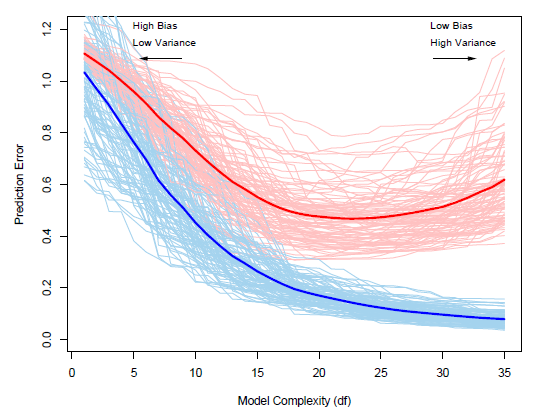• 训练误差（浅蓝色）和测试误差（浅红色）都有波动，并不是一个稳定的值，并且随着模型复杂度的增加，训练误差（浅蓝色）波动越来越小，而测试误差（浅红色）波动则越来越大；
• 随着模型复杂度增加，训练误差（浅蓝色）和平均训练误差（粗蓝线）越来越小，但测试误差（浅红色）和平均测试误差（粗红线）先降低后减小，在相对中间的位置有一个最小值。

1. 为什么训练误差和测试误差会有波动？
2. 训练误差和测试误差的变化趋势说明了什么问题？
3. 造成这种变化趋势的原因是什么？
4. 这种趋势对模型的选择和评价有什么指导意义？

## 1.1 为什么训练误差和测试误差会有波动？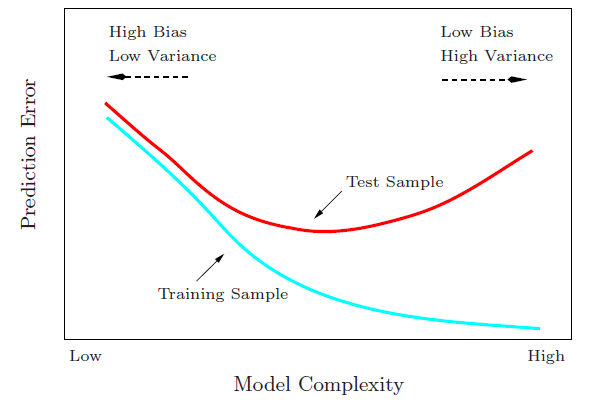## 1.3 造成预测误差变化趋势的原因是什么？

$$\begin{split} \frac{1}{N}\sum^N_{i=1}\text{Err}(x_i) = & \underbrace{\sigma^2_{\epsilon}}_{Irreducible Error} + \underbrace{\frac{1}{N}\sum^N_{i=1}[f(x_i); E\hat{f}(x_i)]^2}_{Ave(Bias^2)} + \underbrace{\frac{p}{N}\sigma^2_{\epsilon}}_{Variance} \\ = & \underbrace{\sigma^2_{\epsilon}}_{Irreducible Error} + \underbrace{\frac{1}{N}\sum^N_{i=1}[f(x_i) ; x_i^T\beta_{*}]^2}_{Ave[Model Bias]^2} + \underbrace{\frac{1}{N}\sum^N_{i=1}[x_i^T\beta_{*} ; Ex_i^T\hat{\beta}]}_{Ave[Estimation Bias]^2} + \underbrace{\frac{p}{N}\sigma^2_{\epsilon}}_{Variance} \end{split}$$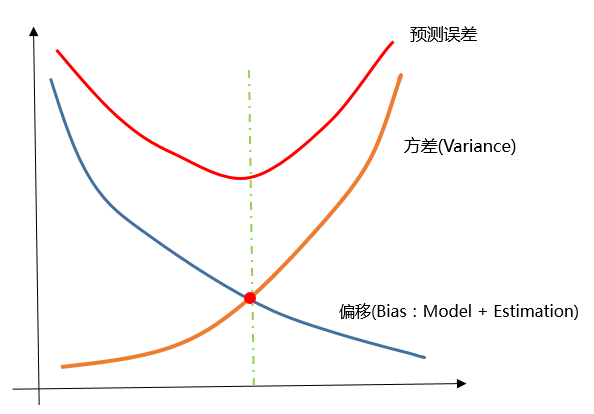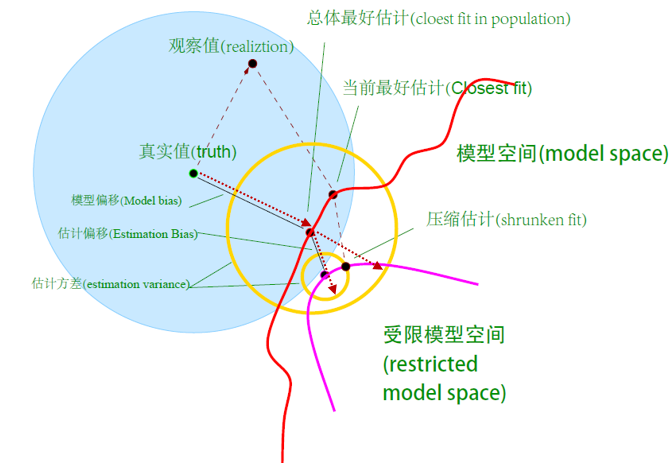## 1.4 对模型选择和评价有什么指导意义？

• 模型选择：根据一组不同复杂度的模型表现，即从某个模型空间中挑选最好的模型；
• 模型评价：选择一个（最好）模型后，在新的数据上来评价其预测误差等评价指标。• 训练集：获得模型及其训练误差，用来训练不同模型；
• 验证集：与训练集相对独立，获取训练模型在该集上的预测误差，用来做模型选择；
• 测试集：与训练集和验证集独立，获得真实的测试误差和其他模型评价指标，用来评价已选择出的模型。

# 2. 模型选择的方法

1. 从训练集划分点数据出来形成验证集来近似测试误差；
2. 对训练误差进行某种转化来近似测试误差。

• 重复抽样与预测稳定性角度：CV、GCV、Boostrap
• 似然与模型复杂度角度：AIC、AICc、BIC、EBIC
• VC维与风险上界控制角度：SRM

## 2.1 重复抽样思路

### CV法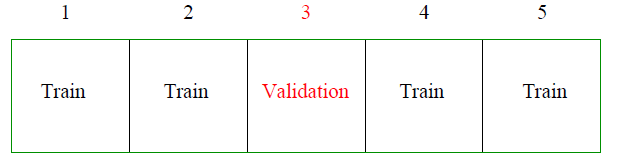$$CV(\hat{f}) = \frac{1}{N}\sum^N_{i=1}L(y_i, \hat{f}^{-\tau(i)}(x_i))$$

$$CV(\hat{f}, \alpha) = \frac{1}{N}\sum^N_{i=1}L(y_i, \hat{f}^{-\tau(i)}(x_i, \alpha))$$

• $$K=N$$时即“留一法（Leave-One-Out, LOO）”，表示每次切分的验证集仅为一个观测，其余的都是训练集，此时新训练集与原始训练集非常接近，则所得的CV值是平均预测误差的近似无偏估计，但是由于这N个训练集相关性也很高，训练的模型则也很相似，而且每次仅评价了一个观测，使得每个验证集上的预测误差相差较大，CV估计的方差较大，另外，N折交叉验证要估计N个模型，计算消耗巨大导致模型选择很慢；
• 如果$$k$$取得较小，比如2，相当于一半数据用来训练一半用来验证，很显然此时新训练集与原始训练集差异较大，则CV值相对于真实平均预测误差会有较大偏误，导致会高估了平均预测误差，不过两个新训练集完全独立，训练出来的两个模型相关性很低，而且验证集数据比较充足，模型评价相对充分，在数据分布没有太大变化的大前提下，两个验证集上的预测误差会比较接近，使得CV估计的方差比较小，而此时计算消耗很少，模型选择效率高。

• 样本量大时，5折交叉验证对预测误差估计便足够，并且计算快速；
• 样本量小时，10折甚至LOO都可以，在损失计算效率的情况下优先考虑预测误差估计的准确性。

• 如果预处理涉及联合使用自变量$$X$$$$Y$$的关系，比如利用$$X$$$$Y$$的相关性等有监督方式来做变量选择，则需要在预处理前就需要对数据进行切分，数据预处理成了模型选择的一部分，而不能先变量筛选，然后在新数据进行交叉验证来做模型选择；
• 如果预处理不涉及自变量$$X$$$$Y$$的关系，仅仅利用$$X$$自身的信息，比如$$X$$的方差或者熵来做变量选择，这种无监督方式的预处理无需对数据进行切分来交叉验证，可以直接先无监督预处理完再利用交叉验证方法来做模型选择。

### Bootstrap法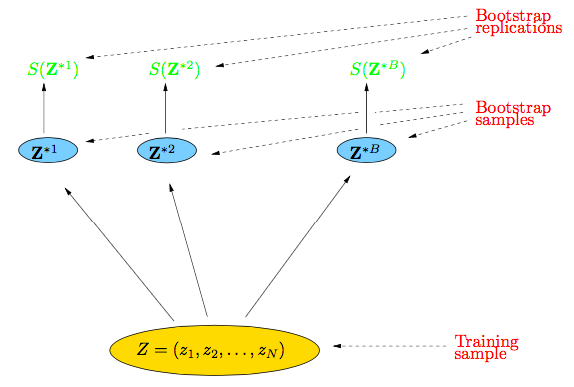bootstrap思想是一种非常重要思想，后来著名的random forest便充分利用了该思路。而且相比目前的数据并行、模型并行的分布式算法思路，我觉得可以从bootstrap抽样角度获取更加稳定的估计量，当然这些都是题外话，与本文话题不相符合，以后可以再谈谈抽样与并行算法之间的感想，实际上都会在“计算效率”与“精度”之间做些权衡。

## 2.2 解析解思路

### 2.2.1 训练误差与样本内误差

$$\text{op} \equiv \text{Err}_{in} ; \bar{err}$$

$$\text{op} \equiv \frac{1}{N} \sum^N_{i=1}[E_{Y^0_i}(Y^0_i ; \hat{y}_i)^2 ; (y_i ; \hat{y}_i)^2)]$$

$$\begin{split} \omega \equiv E_{\mathbf{y}}(\text{op}) & \equiv \frac{1}{N}\sum^N_{i=1}[E_{y_i}E_{Y^0_i}(Y^0_i ; \hat{y}_i)^2 ; E_{y_i}(y_i ; \hat{y}_i)^2] \\ & = \frac{1}{N}\sum^N_{i=1}2(E_{y_i}(y_i \hat{y}_i) ; E_{y_i}y_iE_{y_i}\hat{y}_i) \\ & = \frac{2}{N}\sum^N_{i=1} \text{Cov}(y_i, \hat{y}_i) \end{split}$$

$$E_{\mathbf{y}}(\text{Err}_{in}) = E_{\mathbf{y}}(\bar{err}) + \frac{2}{N}\sum^N_{i=1} \text{Cov}(y_i, \hat{y}_i)$$

$$\sum^N_{i=1}\text{Cov}(y_i, \hat{y}_i) = \sum^N_{i=1}\text{Cov}(y_i, \mathbf{Sy}_i) = \text{trace}(\mathbf{S})\sigma_{\epsilon}^2 = \sum^N_{i=1} d \sigma_{\epsilon}^2$$

### 2.2.2 Cp法与AIC

$$\hat{Err}_{in} = \bar{err} + \hat{\omega}$$

$$C_p= \bar{err} + 2 \cdot \frac{d}{N}\hat{\sigma}_{\epsilon}^2$$

AIC准则与之略有差异，训练误差选用似然函数的负数来代替，而$$\hat{\omega}$$没有噪音方差的估计$$\hat{\sigma}_{\epsilon}^2$$，为如下形式

$$\text{AIC} = -\frac{2}{N}\cdot \text{loglike} + 2 \cdot \frac{d}{N}$$

$$d$$仍是模型的参数个数，用来衡量模型复杂度。对于非线性模型和更复杂的模型，此处$$d$$需要用其他形式来代替。

### 2.2.3 BIC与贝叶斯

BIC准则形式项与AIC很像，同样还是似然负数作为损失，然后加上一个关于自由度与样本相关的项。

$$\text{BIC} = -2 \cdot \text{loglike} + (\log N) \cdot d$$

$$\text{BIC} = \frac{N}{\sigma_{\epsilon}^2}[\bar{err} + (\log N) \cdot \frac{d}{N}\sigma^2_{\epsilon}]$$

$$\begin{split} P(\mathcal{M}_m | \mathbf{X}) & = \frac{P(\mathcal{M}_m) \cdot P(\mathbf{X}|\mathcal{M}_m)}{P(\mathbf{X})} \\ & \propto P(\mathcal{M}_m) \cdot P(\mathbf{X}|\mathcal{M}_m) \\ & = P(\mathcal{M}_m) \cdot \int P(\mathbf{X}|\theta_m, \mathcal{M}_m) P(\theta_m|\mathcal{M}_m) d\theta_m \end{split}$$

$$\frac{P(\mathcal{M}_m | \mathbf{X})}{P(\mathcal{M}_l | \mathbf{X})} = \frac{P(\mathcal{M}_m)}{P(\mathcal{M}_l)} \cdot \frac{P(\mathbf{X}|\mathcal{M}_m)}{P(\mathbf{X}|\mathcal{M}_l)}$$

（注：一句话阐述Laplace技巧即，对于复杂概率函数的似然求解，我们可以将其在参数的极大似然估计处做二阶泰勒展开，根据似然函数在MLE估计处的一阶导为0的性质，原始的概率函数可凑成正态密度函数的形式，于是复杂概率函数就可以用均值和方差均可求的正态分布来近似。）

$$P(\mathcal{M}_m | \mathbf{X} ) = \frac{\exp(-\frac{1}{2}\cdot \text{BIC}_m)}{\sum^M_{l=1}\exp(-\frac{1}{2}\cdot \text{BIC}_l)}$$

EBIC法主要的改进思路是对模型先验分布$$P(\mathcal{M}_m)$$的均匀分布进行合理改进。当变量维数比较大时，含不同变量数的模型个数是不同的，比如$$\mathbf{X}$$现为1000维变量,则包含1个变量的模型$$s$$有1000个，模型集合即为$$S_1$$，而包含2个变量的模型$$s$$$$1000\times 999/2$$，模型集合记为$$S_2$$，可以看到$$S_2$$的大小将近是$$S_1$$的500倍。按照BIC的均匀分布假设，所有模型$$s$$被选择概率相同$$P(s)=1/M$$，这将导致$$P(S_j)$$被选择的概率与之模型集合大小$$\pi(S_j)$$成正比，换句话说，差不多含一半变量数的$$S_{p/2}$$模型集合中的模型$$s$$被选择概率最大，这显然与变量数可能比较小（稀疏）的假设精神相违背，特别在更加高维的情况中，BIC将会更加倾向在较大的模型空间选择，导致选择的变量数过多。

$$\text{EBIC}_{\gamma} = -\log P(\mathbf{X}|\hat{\theta}_m, \mathcal{M}_m) + \frac{d_m}{2}\cdot \log N + \gamma\log \pi(S(\mathcal{M}_m))$$